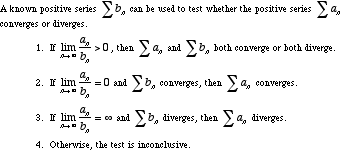index: click on a letter A B C D E F G H I J K L M N O P Q R S T U V W X Y Z A to Z index index: subject areas numbers & symbols sets, logic, proofs geometry algebra trigonometry advanced algebra & pre-calculus calculus advanced topics probability & statistics real world applications multimedia entrieswww.mathwords.com about mathwords website feedback

 Limit Comparison Test A convergence test often used when the terms of a series are rational functions. Essentially, the test determines whether a series is "about as good" as a "good" series or "about as bad" as a "bad" series. The "good" or "bad" series is often a p-series.See also